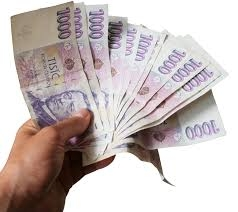# Co-workers 22553

Three co-workers earned a total of CZK 4,720 for repair work. They split so that the first got 20% more than the second and the third got 50% more than the second. How much did everyone get?

a =  1530.8108
b =  1275.6757
c =  1913.5135

### Step-by-step explanation:

a+b+c=4720
a = b+0.20b
c = b+0.50b

a+b+c=4720
a = b+0.20·b
c = b+0.50·b

a+b+c = 4720
a-1.2b = 0
1.5b-c = 0

Row 2 - Row 1 → Row 2
a+b+c = 4720
-2.2b-c = -4720
1.5b-c = 0

Row 3 - 1.5/-2.2 · Row 2 → Row 3
a+b+c = 4720
-2.2b-c = -4720
-1.682c = -3218.182

c = -3218.18181818/-1.68181818 = 1913.51351351
b = -4720+c/-2.2 = -4720+1913.51351351/-2.2 = 1275.67567568
a = 4720-b-c = 4720-1275.67567568-1913.51351351 = 1530.81081081

a = 56640/37 ≈ 1530.810811
b = 47200/37 ≈ 1275.675676
c = 70800/37 ≈ 1913.513514

Our linear equations calculator calculates it.Did you find an error or inaccuracy? Feel free to write us. Thank you!

Tips for related online calculators